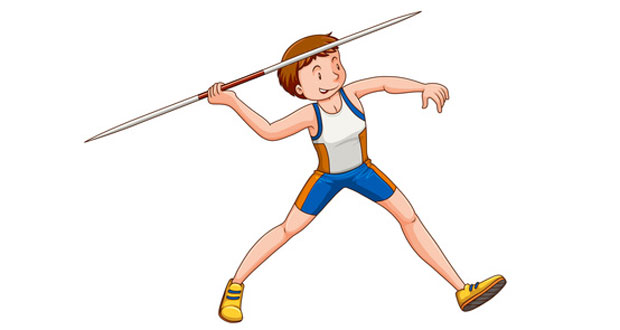# Projectiles In SportA projectile is anybody which is thrown or jumped into the air. Once it has left the ground it will follow a flight path called a parabola until it once more comes back down to earth. This applies to balls, javelins, discus, long jumpers, high jumpers, and horses showjumping. As far as we know there is no escaping the effects of gravity.

Once a body has left the ground there are only two forces acting on it:

• Weight (due to gravity)
• Air resistance

## What can affect the flight path (parabola)?

How the object flies through the air will depend on the air resistance and weight of the object. For example, a heavy object such as a shot put will be virtually unaffected by air resistance. A shuttlecock in badminton is very light and has feathers which increase the air resistance. As a result, it’s flight path is different.

Can you guess what the flight paths of the following might be?

• Table tennis ball
• Cricket ball
• Golf ball
• Javelin

## The equations of motion

Sir Isaac Newton worked out how to calculate the position and speed of objects. He discovered four equations that we can use to calculate everything we need to know about a projectile. However, at this level, we have to assume there is no air resistance when in the real world there might be. The four equations of motion are:

v = u + at

s = 1/( v + u ) t

s = ut + 1/2 a t2

v2 = u2 + 2aS

Where:

• v = final velocity in
• u = initial velocity in metres per second
• s = displacement in metres
• t = time in seconds

When we have a problem involving projectile motion then we simply pick the relevant equation to calculate what we need to know. As mentioned above, air resistance will significantly change the answers in some situations.